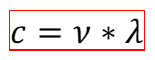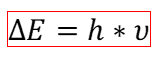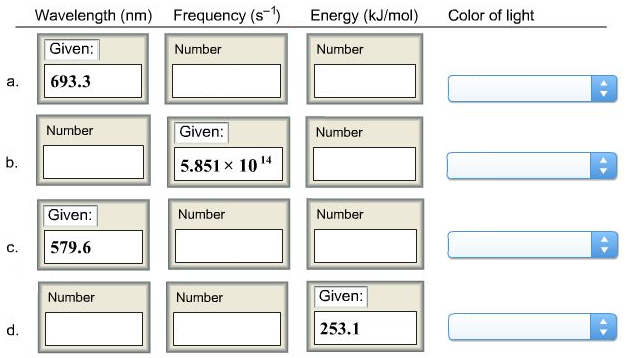# Problem: Complete this table for visible light.

###### FREE Expert Solution

In order to complete each column of the table for visible light, we need the equations that relate each property.

First, frequency (ν) and wavelength (λ) are related to each other through the speed of light (c), with a value of 3x108 m/s:Second, the energy (ΔE) and frequency (ν) are related through Planck’s constant (h), with a value of 6.626x10-34 J*s:Third, the color of the light can be determined more easily from the wavelength, since the visible spectrum ranges from color violet (390 nm), trough blue, green 500 nm), yellow, orange, until red (at 780 nm).

94% (276 ratings)###### Problem Details

Complete this table for visible light.Frequently Asked Questions

What scientific concept do you need to know in order to solve this problem?

Our tutors have indicated that to solve this problem you will need to apply the Electromagnetic Spectrum concept. You can view video lessons to learn Electromagnetic Spectrum. Or if you need more Electromagnetic Spectrum practice, you can also practice Electromagnetic Spectrum practice problems.